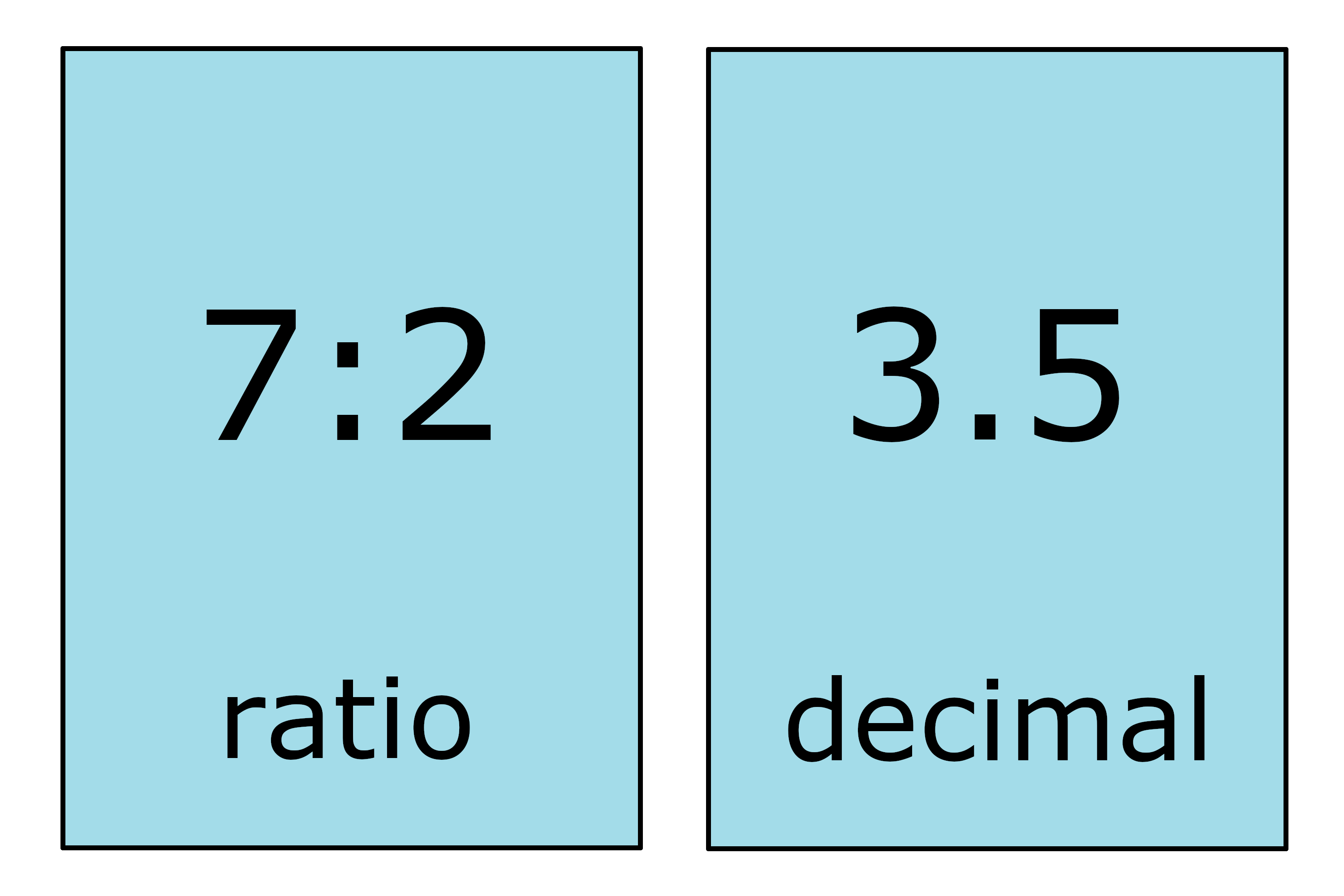# Ratio to Decimal Calculator

Convert a ratio to decimal form by entering both parts below.

Ratio
:

## Decimal:

0.625

### Steps to Convert a Ratio to a Decimal

Step One: Rewrite the ratio as a fraction
5 : 8=58
Step Two: Divide the numerator by the denominator
58=5÷8
5÷8=0.625

## How to Convert a Ratio to a Decimal

There are a few different ways to convert a ratio to a decimal. The easiest way to convert to decimal form is to use a calculator, like the one above. Continue reading, and we’ll show you the steps to do the conversion yourself.

### Step One: Rewrite the Ratio as a Fraction

The first step in converting a ratio to a decimal is to rewrite the ratio as a fraction. To do this, put the first part of the ratio over the second part in fraction form. The first part of the ratio will be the numerator, and the second part will be the denominator.

### Step Two: Solve the Fraction and Express as a Decimal

The final step in the conversion is to solve the fraction to get the decimal value. To do this, divide the numerator by the denominator. The result is the decimal form of the ratio.

For example, convert 7:2 to a decimal value.

Rewrite 7:2 as a fraction.
7:2 = 72

Then solve.
72 = 7 ÷ 2
72 = 3.5

Thus, the decimal value of 7:2 is 3.5Our decimal to ratio calculator can help solve the reverse of this formula.